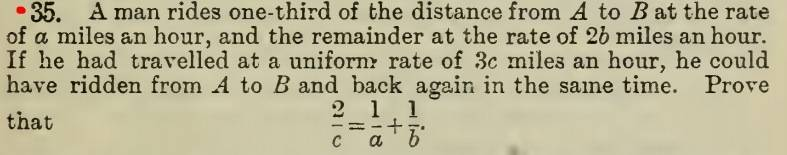# Word problem about distance, rate and time

• paulmdrdo

## Homework StatementD = rt

## The Attempt at a Solution

I let d to be the total distance

d/3a = t1 ----- time taken for 1st part of the trip

d/3b = t2 ----- time taken for 2nd part of the trip

d/3c = t3 ------ time taken for the whole trip with uniform speed

Since t1+t2 =t3

d/3a + d/3b = d/3c

Multiplying both sides by 3abc

bcd + acd = abd

Dividing both sides by d

bc + ac = ab

Factoring out c and diving both sides by it.

1/c = (a + b)/ab = 1/a + 1/b

1/c = 1/a + 1/b not the same as 2/c =1/a + 1/b

Do you think it is just a typo?
[/B]

d/3c = t3 ------ time taken for the whole trip with uniform speed
Hi Paul:

The problems statement says:
"He could have ridden from A to B and back again in the same time."​
Therefore if t3 = time to go from A to B, then t3 = ( t1 + t2) / 2.

Regards,
Buzz

•paulmdrdo
2/c = 1/a +1/b is a typo. It should be 2/c = 1/a +2/b.

Do you think it is just a typo?
No, it's not a typo.

See what Buzz said.